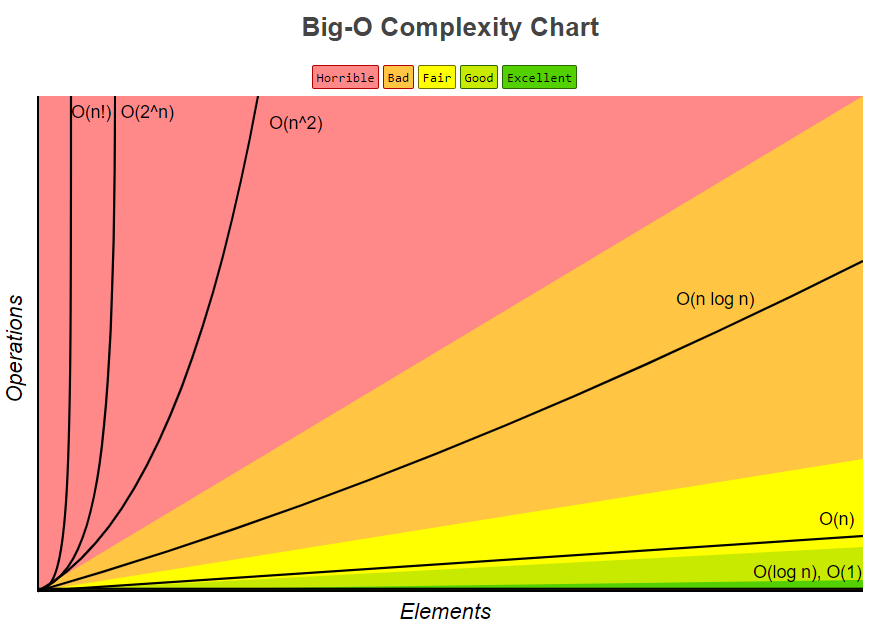Instantly share code, notes, and snippets.

# ThuyNT13/Big_O_Notation.md

Last active August 4, 2022 08:27
Big O Notation

Big O notation is a way for us to describe how long it takes for an algorithm to run. We can use Big O notation to compare how efficient different approaches to solving a problem are. In big O notation we describe the runtime of an algorithm in terms of how quickly the runtime grows as the input to the algorithm gets very, very large. Let’s break down the definition a bit:

• How quickly the runtime grows:

• We can’t give an exact amount of time that an algorithm will run for because it depends on too many things. Is it running on a supercomputer, a really old desktop, or a calculator? Which language is it written in? Is the computer running anything else at the same time? All of these can affect the runtime, so we remove these variables by focusing on how the runtime grows.
• Based on the input:

• Since we are removing runtime from the description we need another way to express the speed - we can’t use seconds anymore. Instead we’ll use the size of the input to describe it. We will use the letter “n” to describe the size of our input.
• As the input becomes very, very large:

• The algorithm might have steps that seem expensive when n is small but are eventually eclipsed by other steps as n grows. Big O analysis is based on the part of the algorithm that grows most quickly as the input grows, so we use very large inputs to tease out this information. This is also known as asymptotic analysis.

One note: If you’ve taken an algorithms class before you may have heard of Big O, Big Theta, and Big Omega - we’re going to use Big O as it’s commonly used in industry: by always trying to offer the tightest description of the runtime.

So what does big O look like? For big O notation we only use the term that grows the most quickly, and we drop any constants.

The simplest and fastest big O term is constant time, written as O(1). This means that the runtime of the algorithm doesn’t scale with the input. For example, the below function doesn’t scale with the input - it accesses and prints the first item whether there are 2 or 2 million items in the array:

```def print_first(array):
print(array)```

If the runtime scales linearly with the input we call it “big O of n” written as O(n). For example, this could be a program that prints each item of a list. As the list gets bigger the runtime gets bigger at the same rate:

```def print_list(array):
for item in array:
print(item)```

We don’t need to describe the rate of growth with constants though. This function is also O(n), not O(2n), even though it runs through the list twice:

```def print_list_twice(array):
for item in array:
print(item)
for item in array:
print(item)```

We only keep the term that grows at the fastest rate. For example, this function runs through the list once and then through the list of n numbers n times O(n+n2), but we’d call it O(n2) because the n2 grows much more quickly than the n:

```def print_list_and_combinations(array):
#print each number
for number in array:
print(number)
#print combinations of numbers
for first_number in array:
for second_number in array:
print(first_number, second_number)```

Common big O notations include (by rate of increase):

• O(1)
• O(log n)
• O(n)
• O(n log n)
• O(n2)
• O(2n)
• O(n!)

Generally we want to minimize the runtime of our algorithms to make them more efficient. Reducing the runtime typically requires the use of additional space though and this cost must be balanced carefully! The graph below shows how the number of operations required scales with the number of elements in the input. Try to avoid high-complexities like O(n!), O(2n), and O(n2) when possible.Graph from http://bigocheatsheet.com/

Gist content by Mary Troiani for presentation she gave at the Algorithms and Technical Interview Study Group - Dec 13, 2017 meetup

This is a part of a series of presentations for Learn Teach Code - Algorithm Study Group